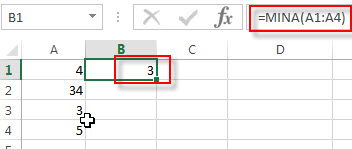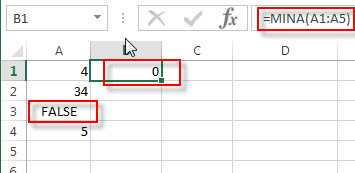# Excel MINA Function

This post will guide you how to use Excel MINA function with syntax and examples in Microsoft excel.

### Description

The Excel MINA function returns the smallest numeric value from the numbers that you provided, while counting text and the logical values (The TRUE value is treated as value 1 and the FALSE value is treated as value 0).

The MIN function is a build-in function in Microsoft Excel and it is categorized as a Statistical Function.

The MIN function is available in Excel 2016, excel 2013, excel 2010, excel 2007, excel 2003, excel XP, excel 2000, Excel 2011 for Mac.

### Syntax

The syntax of the MINA function is as below:

`= MINA (value1,[ value 2], …)`

Where the MINA function arguments are:

• value1 -This is a required argument. A number, or a reference to a number or a range that contains numeric values, text representations of numbers, or logical values
• value2,… – This is an optional argument. A number, or a reference to a number or a range that contains numeric values. It can be up to 255 value argument in Excel 2007 or later, and it only can be up to 30 value argument in Excel 2003.

Note:

• The arguments can be the following values: numbers, arrays or cell reference that contain numbers, text representation of numbers, or logical values (TRUE and FALSE).
• If the argument is an array or reference that contain empty cells or text values, and those values will be ignored.
• The logical TRUE value will be treated as 1; and the logical FALSE value will be treated as 0.
• If an argument that contain error values or text that cannot be converted into numbers and it will return errors.
• If the argument do not want to contain logical values and the text representation of numbers in array or reference, you can use the MIN function.

### Excel MINA Function Examples

The below examples will show you how to use Excel MIN Function to get the smallest value form the numbers you provided in a range.

Example 1:  To get the smallest value from a range A1:A4, just using the following excel formula in Cell B1:

`=MINA(A1:A4)`Example 2:  to get the smallest of the numbers in the range A1:A5 that contain logical FALSE (the value FALSE is evaluated to 0, so it is the smallest value), just using the following excel formula in Cell B1:

`=MINA(A1:A5)`### Related Functions

• Excel MIN function
The Excel MIN function returns the smallest numeric value from the numbers that you provided. Or returns the smallest value in the array.The MIN function is a build-in function in Microsoft Excel and it is categorized as a Statistical Function.The syntax of the MIN function is as below:= MIN(num1,[num2,…numn])….
• Excel MAX function
The Excel MAX function returns the largest numeric value from the numbers that you provided. Or returns the largest value in the array.= MAX(num1,[num2,…numn])…
• Excel MAXA function
The Excel MAXA function returns the largest numeric value from a range of values. The syntax of the MAXA function is as below:= MAXA(value1,[value2,…valueN])…